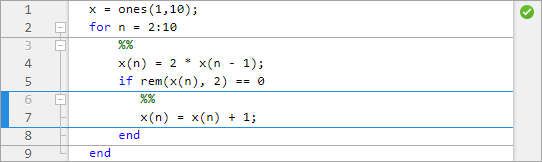## 代码节

### 将您的文件分为多个代码节

MATLAB® 文件通常包含多个命令。您通常一次仅专注于程序中的某个部分，分块操作代码。同样，向其他人解释您的文件时，通常分块介绍您的程序。为帮助介绍这些过程，使用代码节，也称为代码单元或单元模式。代码节包含 MATLAB 脚本中您想要作为一组求值的连续代码行，以两个注释字符 (`%%`) 开头。

• 编辑器选项卡上，点击（或 MATLAB Online™ 中的）。

• 在您想要开始新的代码节的行首输入两个百分比符号 (`%%`)。

`%%` 位于同一行中的文本称为节标题。可以选择是否包含节标题，不过，节标题可提高文件的可读性并在您发布代码时显示为标题。

### 执行代码节

MATLAB Online 中，按钮位于部分中。

MATLAB Online 中，按钮位于部分中。

#### 递增代码节中的值

1. 高亮显示数字或将光标放在数字旁边。

2. 右键点击以打开上下文菜单。

3. 选择递增值和运行节。随即出现一个小型对话框。4./文本框或/文本框中输入合适的值。

5. 点击按钮以在节中增加、减去、乘以或除以所选的数值。

MATLAB 在每次点击后运行该节。

MATLAB 软件不自动保存您对脚本中的数字所做的更改。

### 在文件中的各代码节之间导航

Ctrl + 向上箭头。

MATLAB Online 中，要在各节之间导航，请在编辑器选项卡的导航节中，选择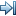转至。然后，从可用选项中进行选择。

### 执行代码节的示例

```edit(fullfile(matlabroot,'help','techdoc','matlab_env',... 'examples','sine_wave.m'))```

1. 在文件的第一行插入分节符和以下标题。

``` %% Calculate and Plot Sine Wave ```

2. `plot(x,y)` 之后插入空白行和第二个分节符。添加节标题 `Modify Plot Properties`，这样整个文件都包含该代码：

```%% Calculate and Plot Sine Wave % Define the range for x. % Calculate and plot y = sin(x). x = 0:1:6*pi; y = sin(x); plot(x,y) %% Modify Plot Properties title('Sine Wave') xlabel('x') ylabel('sin(x)') fig = gcf; fig.MenuBar = 'none';```

3. 保存文件。

4. 将光标放在标题为 `Calculate and Plot Sine Wave` 的节中。在编辑器选项卡上的运行部分中，点击。在 MATLAB Online 中，按钮位于部分中。

随即出现一个显示 `sin(x)` 轨迹图的图窗。5. 对该正弦图进行平滑处理。

1. 高亮显示 `x = 0:1:6*pi; ` 语句中的 `1`

2. 右键点击并选择递增值和运行节。随即出现一个小型对话框。3./文本框中键入 `2`

4. 点击按钮多次。

每次点击后正弦图都会变得更平滑。5. 关闭图窗并保存文件。

6. 运行整个 `sine_wave.m` 文件。带有标题的平滑正弦图显示在新图窗中。### 更改代码节的外观

1. 主页选项卡上，点击环境部分中的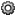此时将显示“预设项”对话框。

2. 在左窗格中，选择 MATLAB > 颜色 > 编程工具

3. 节显示选项下方，选择代码节的外观。您可以选择是否高亮显示节、高亮显示的颜色以及代码节之间是否显示分界线。

### 同时使用代码节与控制语句和函数

MATLAB 根据以下条件自动定义代码块中的节界限：

• MATLAB 在文件顶端和底端插入分节符，创建包含整个文件的代码节。但是，编辑器不会高亮显示包含整个文件的生成节，除非您将一个或多个显式代码节添加到文件中。

• 如果您定义控制流语句（例如 `if``while` 语句）内的分节符，MATLAB 会自动将分节符插入包含语句开始和结束的行。

• 如果您在函数内定义分节符，MATLAB 会将分节符插入函数声明和函数结束语句中。如果您没有用 `end` 语句结束函数，MATLAB 将视该函数的结尾紧发生在下个函数的开头前。

#### 嵌套的代码节分节符

```t = 0:.1:pi*4; y = sin(t); for k = 3:2:9 %% y = y + sin(k*t)/k; if ~mod(k,3) %% display(sprintf('When k = %.1f',k)); plot(t,y) end end```

• 在嵌套的最外层，一个节涵盖整个文件。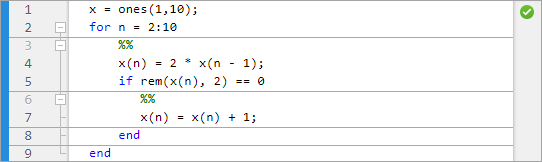只有您在代码块内的同一级别指定分节符，MATLAB 才会在该代码块内定义节。因此，MATLAB 将该光标视为位于包含整个文件的节内。

• 在嵌套的第二级，一个节位于 `for` 循环内。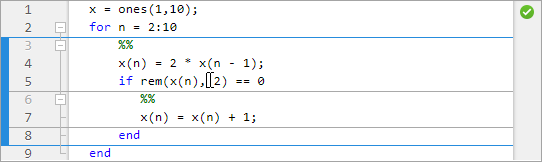• 在嵌套的第三级，一个节位于 `if` 语句内。